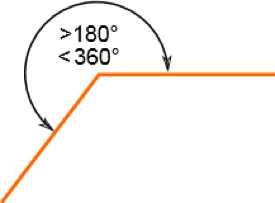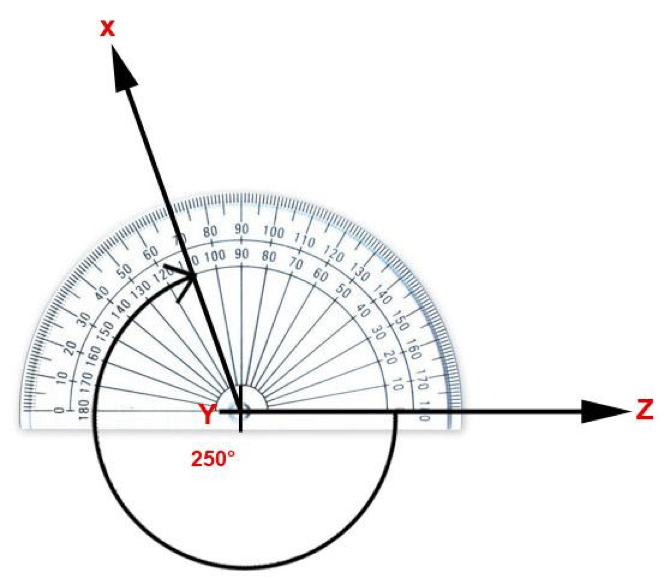# What are Reflex Angles?

A reflex angle is the larger angle, it’s always more than 180° (half a circle) but less than 360°  (A full circle). It’s possibly the most confusing of the angles because it’s always on the outside.# Reflex Angles explained

There are six types of angle in total;

An Acute angle is the smallest, measuring more than 0° but less than 90°.

Next up is a Right angle, also taught as a quarter turn. This angle always measures 90 °.

An Obtuse angle measures more than 90° but less than 180°.

A Straight angle or a half turn is always 180 °.

Reflex is the next largest measuring more than 180° but less than 360°.

We then have a full rotation or full circle at 360°.

# Angle Family

If you struggle to remember the different names of the angles, try watching this video. The angles are introduced as different characters within a family which might help you to remember!

# How do you measure a Reflex Angle?

First, make sure that it is the reflex angle you’re being asked to measure! Lots of children mistakenly, but very carefully measure the wrong angle. Usually, the angle you’re being asked to measure will have a little circle drawn around the point to show you which side needs measuring.You can measure the reflex angle in one of two ways; either measure the inner angle and subtract it from 360° (to give you the measure of the reflex angle) or measure the reflex angle itself.

A reflex angle is probably the trickiest angle to measure because it will be larger than your standard 180° protractor so you’ll need to do a bit of accurate drawing.

1. Line up your protractor with one side of the angle (in this case y to z), make sure that the centre of the protractor lines up with the centre point of where the two lines meet. (N.B Lots of children use the straight edge of the protractor instead of the line). Make sure that your line goes through 0 °.2. Make a small mark on your paper to show where 180° is. If you want to, you could even draw a faint line to show the 180° line.

3.You now need to measure the remainder. Using the outside scale on your protractor, measure the angle from the 180° line you have drawn to the second line you have been given (in this case y to x).

4. Add together 180° to the second measurement and this will be your reflex angle.

5. If you want to check your answer, measure the inside angle and check that the two measurements added together equal 360°.

## How do you measure a Reflex Angle – Video explanation?

If you're a visual learner and would prefer to watch, have a look at this video link;

# Worksheets and Practice

EdPlace have loads of great worksheets to teach you about angles. We’ve listed a few of our favourites below:

Year 5 – Angle multiples of 90°.

Year 5 – Geometry – Estimate angles.

Year 5 – Geometry – Calculate missing angles at a point

Year 6 – Geometry – What’s the angle?

Year 6 – Geometry – Angles at a point.

Year 6 – Triangles – Calculate unknown angles

# Further Learning

If you enjoy geometry and want to give yourself a challenge, why not try some of the puzzles and problems set by the NRich team from the University of Cambridge?

Click the link below to try out a selection of puzzles linked to angles, triangles and reflex angles to really get you thinking!

https://nrich.maths.org/public/leg.php?code=77